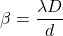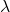Question

Monochromatic coherent light shines through a pair of slits. If the distance between these slits is decreased, which of the following statements are true of the resulting interference pattern?A) The distance between the maxima stays the same.
B) The distance between the maxima decreases.
C) The distance between the minima stays the same.
D) The distance between the minima increases.
E) The distance between the maxima increases.

1.thienan

The correct statements are D and E.

Explanation:

The fringe width is given by the following formula as :Here,is wavelength of light

D is distance between slit and the screen

d is slit width.

If the between these slits is decreased, the fringe width increases. As a result, the distance between the minima increases and also the distance between the maxima increases.